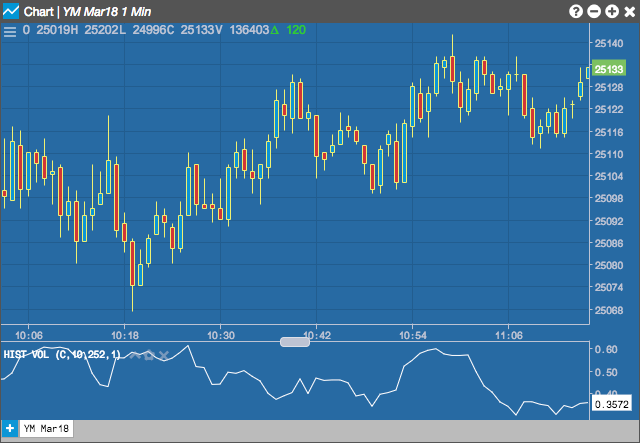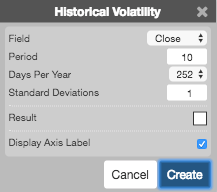Charts

# Historical Volatility (HV)

Historical Volatility is just a standard deviation of the underlying percent return over n-periods.## Configuration Options• Field: Price or combination of prices to use as the base for average calculations. Possible values include:
• Open
• High
• Low
• Close
• HL/2 $$\left ( \frac{High + Low}{2} \right )$$
• HLC/3 $$\left ( \frac{High + Low + Close}{3} \right )$$
• HLCC/4 $$\left ( \frac{High + Low + Close + Close}{4} \right )$$
• OHLC/4 $$\left ( \frac{Open + High + Low + Close}{4} \right )$$
• Period: Number of bars to use in the calculations.
• Days Per Year: Whether to base the calculations on the numberr of calendar days or trading days in the year.
• Standard Deviations: Number of standard deviations to deviate from the average.
• Color Selectors: Colors to use for graph elements.
• Display Axis Label: Whether to display the most recent value on the Y axis.

## Formula

$HV = \sigma = \sqrt{\frac{\sum_{i=1}^{n}(r_{i}-m)^{2}}{(n-1)}}$

where

$\sigma = Standard\;deviation$

$r_{i} = \frac{P_{t}-P_{t-1}}{P_{t}}$

$m = MA\;or\;average\;of\;all\;data\;points$

$n = Number\;of\;data\;points$